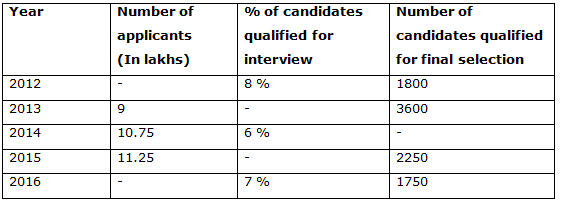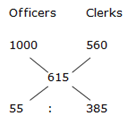# IBPS PO Mains 2018 – Quantitative Aptitude Questions Day-11

Dear Readers, Bank Exam Race for the Year 2018 is already started, To enrich your preparation here we have providing new series of Practice Questions on Quantitative Aptitude – Section. Candidates those who are preparing for IBPS PO Mains 2018 Exams can practice these questions daily and make your preparation effective.

[WpProQuiz 4076]

1) 4 years ago, the ratio of ages of Rajiv and Ganga is 5: 4. Vaishali is 3 years younger than Rajiv and 2 years elder than Ganga. Find the sum of the present age of Rajiv and Ganga?

a) 57 years

b) 62 years

c) 53 years

d) 60 years

e) None of these

2) The circumference of a circle is half of the perimeter of a rectangle. The area of the circle is 1386 Sq m. What is the area of the rectangle if the breadth of the rectangle is 50 m?

a) 4100 Sq m

b) 4350 Sq m

c) 3600 Sq m

d) 4800 Sq m

e) None of these

3) Ajay and Jega enter into a partnership by investing Rs. 40000 and Rs. 60000 respectively. At the end of 5 months, Ajay withdraw one – fourth of his initial investment and at the end of 7 months, Jega withdraw two-third of his initial investment and then Saaru entered with a capital of Rs. 45000. If the total profit at the end of the year is Rs. 173250, then find the share of Jega?

a) Rs. 66000

b) Rs. 78000

c) Rs. 82000

d) Rs. 54000

e) None of these

4) The average salary of the whole employees in a company is Rs. 615 per day. The average salary of officers is Rs. 1000 per day and that of clerks is Rs. 560 per day. If the number of officers is 20, then find the number of clerks in the company?

a) 120

b) 130

c) 160

d) 140

e) None of these

5) Samir invested Rs. 30000 in two different parts one at 6% per annum for CI (Compounded half yearly) and another one at 10% SI. At the end of the year, he received total interest Rs. 2218, then find the amount invested in SI?

a) Rs. 15000

b) Rs. 10000

c) Rs. 12000

d) Rs. 18000

e) None of these

Directions (Q. 6 – 10) Study the following information carefully and answer the given questions.

The following table shows the total number of candidates applied for SBI PO and the percentage of candidates qualified for interview and total number of candidates qualified for final selection in different years. Some data’s are missing here. We have to find the missing data according to the question given.6) Find the difference between the number of candidates qualified for the interview in the year 2012 to that in the year 2014, if the ratio of total number of applicants in the year 2012 to that of 2013 is 5 : 6?

a) 5200

b) 4500

c) 3800

d) 6600

e) None of these

7) Find the total number of candidates applied for the post of SBI PO in the year 2016, if the percentage of total number of candidates qualified for final selection after interview in the year 2016 is 2 %?

a) 10.5 lakhs

b) 13 lakhs

c) 8.5 lakhs

d) 5 lakhs

e) None of these

8) Find the ratio between the total number of candidates qualified for final selection in the year 2014 to that of 2015, if the percentage of total number of candidates qualified for final selection after interview in the year 2014 is 5 %?

a) 43: 30

b) 35: 18

c) 24: 19

d) 37: 22

e) None of these

9) Find the total number of candidates qualified for the interview in the year 2014, 2015 and 2016 together, if the total number of applicants in the year 2013 is 72 % of total number of applicants in the year 2016 and the percentage of candidates qualified for the interview in the year 2015 is 5%?

a) 215600

b) 237850

c) 208250

d) 192400

e) None of these

10) Find the average number of candidates qualified for the final selection in all the given years together, if the percentage of total number of candidates qualified for final selection after interview in the year 2014 is 5%?

a) 2850

b) 2525

c) 2375

d) 2400

e) None of these

4 years ago, the ratio of ages of Rajiv and Ganga = 5: 4 (5x, 4x)

Present ages of Rajiv and Ganga = 5x + 4, 4x + 4

Vaishali = Rajiv – 3

Vaishali = Ganga + 2

Rajiv – 3 = Ganga + 2

5x + 4 – 3 = 4x + 4 + 2

5x + 1 = 4x + 6

X = 5

Present age of Rajiv = 29 years

Present age of Ganga = 24 years

The sum of the present age of Rajiv and Ganga = 29 + 24 = 53 years

Area of a circle = πr2

1386 = (22/7)*r2

1386*(7/22) = r2

r2 = 441

r = 21 m

Circumference = 2πr = 2*(22/7)*21 = 132 m

Perimeter of the rectangle = 2*132 = 264 m

264 = 2*(l + 50)

132 = l + 50

l = 132 – 50

l = 82

Area of the rectangle = 82*50= 4100 Sq m

The share of Ajay, Jega and Saaru

= > [40000*5 + 40000*(3/4)*7] : [60000*7 + 60000*(1/3)*5] : [45000*5]

= > 410000 : 520000 : 225000

= > 82 : 104 : 45

Total profit = Rs. 173250

231’s = 173250

1’s = 750

The share of Jega = 104* 750 = Rs. 78000

Let the number of clerks in the company be x,

1000*20 + 560*x = 615*(20 + x)

20000 + 560x = 12300 + 615x

7700 = 55x

X = 7700/55 = 140

Total number of clerks in the company = 140

Shortcuts:= > 1: 7

1’s = 20

Total number of clerks in the company = 7’s = 140

Let us take one part be x and another one be 30000 – x,

According to the question,

X*[(1 + 6/200)2 – 1) + [(30000 – x)*10*1]/100 = 2218

X*[(206/200)2 – 1)] + 3000 – (10x/100) = 2218

(609x/10000) + 3000 – (10x/100) = 2218

(609x/10000) – (10x/100) = 2218 – 3000

-391x/10000 = – 782

X = 782*(10000/391) = 20000

The amount invested in S.I = 30000 – x = 30000 – 20000 = Rs. 10000

Direction (6-10) :

The ratio of total number of applicants in the year 2012 to that of 2013 = 5 : 6

6’s = 900000

1’s = 150000

The total number of applicants in the year 2012 = 5’s = 750000

The number of candidates qualified for the interview in the year 2012

= > 750000*(8/100) = 60000

The number of candidates qualified for the interview in the year 2014

= > 1075000*(6/100) = 64500

Required difference = 64500 – 60000 = 4500

The percentage of total number of candidates qualified for final selection after interview in the year 2016 = 2 %

The total number of candidates applied for the post of SBI PO in the year 2016

= > x*(7/100)*(2/100) = 1750

= > x = 1750*(100/7)*(100/2)

= > x = 1250000 = 12.5 lakhs

The percentage of total number of candidates qualified for final selection after interview in the year 2014 = 5 %

The total number of candidates qualified for final selection in the year 2014

= > 1075000*(6/100)*(5/100) = 3225

The total number of candidates qualified for final selection in the year 2015

= > 2250

Required ratio = 3225: 2250 = 43: 30

The percentage of candidates qualified for the interview in the year 2015 = 5%

The total number of applicants in the year 2013 = 72 % of total number of applicants in the year 2016

900000 = (72/100)* the total number of applicants in the year 2016

The total number of applicants in the year 2016

= > 900000*(100/72) = 1250000

The total number of candidates qualified for the interview in the year 2014, 2015 and 2016 together

= >1075000*(6/100) + 1125000*(5/100) + 1250000*(7/100)

= > 64500 + 56250 + 87500 = 208250

The percentage of total number of candidates qualified for final selection after interview in the year 2014 = 5 %

The total number of candidates qualified for final selection in the year 2014

= > 1075000*(6/100)*(5/100) = 3225

The total number of candidates qualified for the final selection in all the given years together

= > 1800 + 3600 + 3225 + 2250 + 1750 = 12625

Required average = 12625/5 = 2525

Daily Practice Test Schedule | Good Luck

 Topic Daily Publishing Time Daily News Papers & Editorials 8.00 AM Current Affairs Quiz 9.00 AM Current Affairs Quiz (Hindi) 9.30 AM IBPS PO Mains – Reasoning 10.00 AM IBPS PO Mains– Quantitative Aptitude 11.00 PM Vocabulary (Based on The Hindu) 12.00 PM IBPS PO Mains – English Language 1.00 PM IBPS PO/Clerk – GK 3.00 PM Daily Current Affairs Updates 5.00 PM IBPS Clerk Prelims – Reasoning 6.00 PM IBPS Clerk Prelims – Reasoning (Hindi) 6.30 PM IBPS Clerk Prelims – Quantitative Aptitude 7.00 PM IBPS Clerk Prelims – Quantitative Aptitude (Hindi) 7.30 PM IBPS Clerk Prelims – English Language 8.00 PM